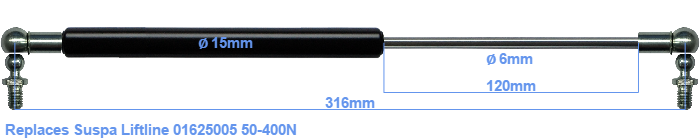# Replacement for Suspa Liftline 01625005 50-400N

£27.29 (excl. VAT)

Replacement gas spring for the Suspa Liftline 01625005 50-400 Newton. This fits a (possibly already present) ball with a diameter of 10mm. A corresponding ball is included. Brand: Hahn.
 Force Choose an option50 Newton70 Newton75 Newton90 Newton100 Newton110 Newton125 Newton130 Newton150 Newton170 Newton175 Newton190 Newton200 Newton210 Newton225 Newton230 Newton250 Newton270 Newton275 Newton290 Newton300 Newton310 Newton325 Newton330 Newton350 Newton370 Newton375 Newton390 Newton400 NewtonClear
This gas spring is also known as 16-1 016 25005, 16-1-154-124-A246-B246.